6.11.0.4

### VIAccumulators

When you ask ISL+ to apply some function f to an argument a, you usually get some value v. If you evaluate (f a) again, you get v again. As a matter of fact, you get v no matter how often you request the evaluation of (f a).The function application may also loop forever or signal an error, but we ignore these possibilities. We also ignore random, which is the true exception to this rule. Whether the function is applied for the first time or the hundredth time, whether the application is located in DrRacket’s interactions area or inside the function itself, doesn’t matter. The function works according to its purpose statement, and that’s all you need to know.

This principle of context-independence plays a critical role in the design of recursive functions. When it comes to design, you are free to assume that the function computes what the purpose statement promises—even if the function isn’t defined yet. In particular, you are free to use the results of recursive calls to create the code of some function, usually in one of its cond clauses. The template and coding steps of the design recipes for both structurally and generative-recursive functions rely on this idea.

While context-independence facilitates the design of functions, it causes two problems. In general, context-independence induces a loss of knowledge during a recursive evaluation; a function does not “know” whether it is called on a complete list or on a piece of that list. For structurally recursive programs, this loss of knowledge means that they may have to traverse data more than once, inducing a performance cost. For functions that employ generative recursion, the loss means that the function may not be able to compute the result at all. The preceding part illustrates this second problem with a graph traversal function that cannot find a path between two nodes for a circular graph.

This part introduces a variant of the design recipes to address this “loss of context” problem. Since we wish to retain the principle that (f a) returns the same result no matter how often or where it is evaluated, the only solution is to add an argument that represents the context of the function call. We call this additional argument an accumulator. During the traversal of data, the recursive calls continue to receive regular arguments while accumulators change in relation to those and the context.

Designing functions with accumulators correctly is clearly more complex than any of the design approaches from the preceding chapters. The key is to understand the relationship between the proper arguments and the accumulators. The following chapters explain how to design functions with accumulators and how they work.

### 31The Loss of Knowledge

Both functions designed according to structural recipes and the generative one suffer from the loss of knowledge, though in different ways. This chapter explains with two examples—one from each category—how the lack of contextual knowledge affects the performance of functions. While the first section is about structural recursion, the second one addresses concerns in the generative realm.

#### 31.1A Problem with Structural Processing

Sample Problem You are working for a geometer team that will measure the length of road segments. The team asked you to design a program that translates these relative distances between a series of road points into absolute distances from some starting point.

For example, we might be given a line such as this: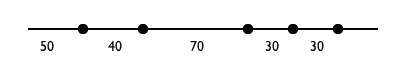Each number specifies the distance between two dots. What we need is the following picture, where each dot is annotated with the distance to the left-most end: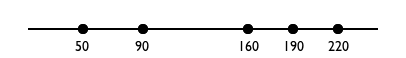Designing a program that performs this calculation is a mere exercise in structural function design. Figure 177 contains the complete program. When the given list is not '(), the natural recursion computes the absolute distance of the remainder of the dots to the first one on (rest l). Because the first is not the actual origin and has a distance of (first l) to the origin, we must add (first l) to each number on the result of the natural recursion. This second step—adding a number to each item on a list of numbers—requires an auxiliary function.

 ; [List-of Number] -> [List-of Number] ; converts a list of relative to absolute distances ; the first number represents the distance to the origin (check-expect (relative->absolute '(50 40 70 30 30)) '(50 90 160 190 220)) (define (relative->absolute l) (cond [(empty? l) '()] [else (local ((define rest-of-l (relative->absolute (rest l))) (define adjusted (add-to-each (first l) rest-of-l))) (cons (first l) adjusted))])) ; Number [List-of Number] -> [List-of Number] ; adds n to each number on l (check-expect (cons 50 (add-to-each 50 '(40 110 140 170))) '(50 90 160 190 220)) (define (add-to-each n l) (cond [(empty? l) '()] [else (cons (+ (first l) n) (add-to-each n (rest l)))]))

Figure 177: Converting relative distances to absolute distances

While designing the program is relatively straightforward, using it on larger and larger lists reveals a problem. Consider the evaluation of the following expression:

As we increase size, the time needed grows even faster:

 size 1000 2000 3000 4000 5000 6000 7000 time 25 109 234 429 689 978 1365
Instead of doubling as we go from 1000 to 2000 items, the time quadruples. This is also the approximate relationship for going from 2000 to 4000, and so on.The times will differ from computer to computer and year to year. These measurements were conducted in 2017 on a MacMini running OS X 10.11; the previous measurement took place in 1998, and the times were 100x larger. Using the terminology of Intermezzo 5: The Cost of Computation, we say that the function’s performance is O(n2) where n is the length of the given list.

Exercise 489. Reformulate add-to-each using map and lambda.

Exercise 490. Develop a formula that describes the abstract running time of relative->absolute. Hint Evaluate the expression

by hand. Start by replacing size with 1, 2, and 3. How many recursions of relative->absolute and add-to-each are required each time?

Considering the simplicity of the problem, the amount of work that the program performs is surprising. If we were to convert the same list by hand, we would tally up the total distance and just add it to the relative distances as we take steps along the line. Why can’t a program do so?

Let’s attempt to design a version of the function that is close to our manual method. We still start from the list-processing template:
 (define (relative->absolute/a l) (cond [(empty? l) ...] [else (... (first l) ... ... (relative->absolute/a (rest l)) ...)]))
Now let’s simulate a hand-evaluation:
 (relative->absolute/a (list 3 2 7)) == (cons ... 3 ... (relative->absolute/a (list 2 7))) == (cons ... 3 ... (cons ... 2 ... (relative->absolute/a (list 7)))) == (cons ... 3 ... (cons ... 2 ... (cons ... 7 ... (relative->absolute/a '()))))
The first item of the result list should obviously be 3, and it is easy to construct this list. But, the second one should be (+ 3 2), yet the second instance of relative->absolute/a has no way of “knowing” that the first item of the original list is 3. The “knowledge” is lost.

Again, the problem is that recursive functions are independent of their context. A function processes L in (cons N L) the same way as in (cons K L). Indeed, if given L by itself, it would also process the list in that way.

To make up for the loss of “knowledge,” we equip the function with an additional parameter: accu-dist. The latter represents the accumulated distance, which is the tally that we keep when we convert a list of relative distances to a list of absolute distances. Its initial value must be 0. As the function traverses the list, it must add its numbers to the tally.

Here is the revised definition:
 (define (relative->absolute/a l accu-dist) (cond [(empty? l) '()] [else (local ((define tally (+ (first l) accu-dist))) (cons tally (relative->absolute/a (rest l) tally)))]))
The recursive application consumes the rest of the list and the new absolute distance of the current point to the origin. Although both arguments are changing for every call, the change in the second one strictly depends on the first argument. The function is still a plain list-processing procedure.

Now let’s evaluate our running example again:
 (relative->absolute/a (list 3 2 7)) == (relative->absolute/a (list 3 2 7) 0) == (cons 3 (relative->absolute/a (list 2 7) 3)) == (cons 3 (cons 5 (relative->absolute/a (list 7) 5))) == (cons 3 (cons 5 (cons 12 ???))) == (cons 3 (cons 5 (cons 12 '())))
Stop! Fill in the question marks in line 4.

The hand-evaluation shows just how much the use of an accumulator simplifies the conversion process. Each item in the list is processed once. When relative->absolute/a reaches the end of the argument list, the result is completely determined and no further work is needed. In general, the function performs on the order of N natural recursion steps for a list with N items.

One problem is that, unlike relative->absolute, the new function consumes two arguments, not just one. Worse, someone might accidentally misuse relative->absolute/a by applying it to a list of numbers and a number that isn’t 0. We can solve both problems with a function definition that uses a local definition to encapsulate relative->absolute/a; figure 178 shows the result. Now, relative->absolute is indistinguishable from relative->absolute.v2 with respect to input-output.

 ; [List-of Number] -> [List-of Number] ; converts a list of relative to absolute distances ; the first number represents the distance to the origin (check-expect (relative->absolute.v2 '(50 40 70 30 30)) '(50 90 160 190 220)) (define (relative->absolute.v2 l0) (local ( ; [List-of Number] Number -> [List-of Number] (define (relative->absolute/a l accu-dist) (cond [(empty? l) '()] [else (local ((define accu (+ (first l) accu-dist))) (cons accu (relative->absolute/a (rest l) accu)))]))) (relative->absolute/a l0 0)))

Figure 178: Converting relative distances with an accumulator

Now let’s look at how this version of the program performs. To this end, we evaluate

and tabulate the results for several values of size:

 size 1000 2000 3000 4000 5000 6000 7000 time 0 0 0 0 0 1 1
Amazingly, relative->absolute.v2 never takes more than one second to process such lists, even for a list of 7000 numbers. Comparing this performance to the one of relative->absolute, you may think that accumulators are a miracle cure for all slow-running programs. Unfortunately, this isn’t the case, but when a structurally recursive function has to re-process the result of the natural recursion you should definitely consider the use of accumulators. In this particular case, the performance improved from O(n2) to O(n)with an additional large reduction in the constant.

Exercise 491. With a bit of design and a bit of tinkering, a friend of yours came up with the following solution for the sample problem:Adrian German and Mardin Yadegar suggested this exercise.
 (define (relative->absolute l) (reverse (foldr (lambda (f l) (cons (+ f (first l)) l)) (list (first l)) (reverse (rest l)))))
This simple solution merely uses well-known ISL+ functions: reverse and foldr. Using lambda, as you know, is just a convenience. You may also recall from Abstraction that foldr is designable with the design recipes presented in the first two parts of the book.

Does your friend’s solution mean there is no need for our complicated design in this motivational section? For an answer, see Recognizing the Need for an Accumulator, but reflect on the question first. Hint Try to design reverse on your own.

#### 31.2A Problem with Generative Recursion

Let’s revisit the problem of “traveling” along a path in a graph:

Sample Problem Design an algorithm that checks whether two nodes are connected in a simple graph. In such a graph, each node has exactly one, directional connection to another, and possibly itself.

Algorithms that Backtrack covers the variant where the algorithm has to discover the path. This sample problem is simpler than that because this section focuses on the design of an accumulator version of the algorithm.

Consider the sample graph in figure 179. There are six nodes, A through F, and six connections. A path from A to E must contain B and C. There is no path, though, from A to F or from any other node besides itself.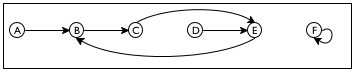(define a-sg '((A B) (B C) (C E) (D E) (E B) (F F)))

Figure 179: A simple graph

The right part of figure 179 shows how to represent this graph with nested lists. Each node is represented by a list of two symbols. The first symbol is the label of the node; the second one is the single node that is reachable from the first one. Here are the relevant data definitions:
 ; A SimpleGraph is a [List-of Connection] ; A Connection is a list of two items: ;   (list Node Node) ; A Node is a Symbol.
They are straightforward translations of our informal descriptions.

We already know that the problem calls for generative recursion, and it is easy to create the header material:
 ; Node Node SimpleGraph -> Boolean ; is there a path from origin to destination ; in the simple graph sg (check-expect (path-exists? 'A 'E a-sg) #true) (check-expect (path-exists? 'A 'F a-sg) #false) (define (path-exists? origin destination sg) #false)
What we need are answers to the four basic questions of the recipe for generative recursion:
• The problem is trivial if origin is the same as destination.

• The trivial solution is #true.

• If origin is not the same as destination, there is only one thing we can do: step to the immediate neighbor and search for destination from there.

• There is no need to do anything if we find the solution to the new problem. If origin’s neighbor is connected to destination, then so is origin. Otherwise there is no connection.

From here we just need to express these answers in ISL+ to obtain a full-fledged program.

 ; Node Node SimpleGraph -> Boolean ; is there a path from origin to destination in sg (check-expect (path-exists? 'A 'E a-sg) #true) (check-expect (path-exists? 'A 'F a-sg) #false) (define (path-exists? origin destination sg) (cond [(symbol=? origin destination) #t] [else (path-exists? (neighbor origin sg) destination sg)])) ; Node SimpleGraph -> Node ; determine the node that is connected to a-node in sg (check-expect (neighbor 'A a-sg) 'B) (check-error (neighbor 'G a-sg) "neighbor: not a node") (define (neighbor a-node sg) (cond [(empty? sg) (error "neighbor: not a node")] [else (if (symbol=? (first (first sg)) a-node) (second (first sg)) (neighbor a-node (rest sg)))]))

Figure 180: Finding a path in a simple graph

Figure 180 contains the complete program, including the function for finding the neighbor of a node in a simple graph—a straightforward exercise in structural recursion—and test cases for both possible outcomes. Don’t run the program, however. If you do, be ready with your mouse to stop the run-away program. Indeed, even a casual look at the function suggests that we have a problem. Although the function is supposed to produce #false if there is no path from origin to destination, the program doesn’t contain #false anywhere. Conversely, we need to ask what the function actually does when there is no path between two nodes.

Take another look at figure 179. In this simple graph there is no path from C to D. The connection that leaves C passes right by D and instead goes to E. So let’s look at a hand-evaluation:
 (path-exists? 'C 'D '((A B)(F F))) == (path-exists? 'E 'D '((A B)(F F))) == (path-exists? 'B 'D '((A B)(F F))) == (path-exists? 'C 'D '((A B)(F F)))
It confirms that as the function recurs, it calls itself with the exact same arguments again and again. In other words, the evaluation never stops.

Our problem with path-exists? is again a loss of “knowledge,” similar to that of relative->absolute above. Like relative->absolute, the design of path-exists? uses a recipe and assumes that recursive calls are independent of their context. In the case of path-exists? this means, in particular, that the function doesn’t “know” whether a previous application in the current chain of recursions received the exact same arguments.

The solution to this design problem follows the pattern of the preceding section. We add a parameter, which we call seen and which represents the accumulated list of starter nodes that the function has encountered, starting with the original application. Its initial value must be '(). As the function checks on a specific origin and moves to its neighbors, origin is added to seen.

Here is a first revision of path-exists?, dubbed path-exists?/a:
 ; Node Node SimpleGraph [List-of Node] -> Boolean ; is there a path from origin to destination ; assume there are no paths for the nodes in seen (define (path-exists?/a origin destination sg seen) (cond [(symbol=? origin destination) #true] [else (path-exists?/a (neighbor origin sg) destination sg (cons origin seen))]))
The addition of the new parameter alone does not solve our problem, but, as the hand-evaluation of

(path-exists?/a 'C 'D '((A B)(F F)) '())

shows, it provides the foundation for one:
 == (path-exists?/a 'E 'D '((A B)(F F)) '(C)) == (path-exists?/a 'B 'D '((A B)(F F)) '(E C)) == (path-exists?/a 'C 'D '((A B)(F F)) '(B E C))
In contrast to the original function, the revised function no longer calls itself with the exact same arguments. While the three arguments proper are again the same for the third recursive application, the accumulator argument is different from that of the first application. Instead of '(), it is now '(B E C). The new value tells us that during the search of a path from 'C to 'D, the function has inspected 'B, 'E, and 'C as starting points.

All we need to do now is to make the algorithm exploit the accumulated knowledge. Specifically, the algorithm can determine whether the given origin is already an item in seen. If so, the problem is also trivially solvable, yielding #false as the solution. Figure 181 contains the definition of path-exists.v2?, which is the revision of path-exists?. The definition refers to member?, an ISL+ function.

 ; Node Node SimpleGraph -> Boolean ; is there a path from origin to destination in sg (check-expect (path-exists.v2? 'A 'E a-sg) #true) (check-expect (path-exists.v2? 'A 'F a-sg) #false) (define (path-exists.v2? origin destination sg) (local (; Node Node SimpleGraph [List-of Node] -> Boolean (define (path-exists?/a origin seen) (cond [(symbol=? origin destination) #t] [(member? origin seen) #f] [else (path-exists?/a (neighbor origin sg) (cons origin seen))]))) (path-exists?/a origin '())))

Figure 181: Finding a path in a simple graph with an accumulator

The definition of path-exists.v2? also eliminates the two minor problems with the first revision. By localizing the definition of the accumulating function, we can ensure that the first call always uses '() as the initial value for seen. And, path-exists.v2? satisfies the exact same signature and purpose statement as the path-exists? function.

Still, there is a significant difference between path-exists.v2? and relative-to-absolute2. Whereas the latter was equivalent to the original function, path-exists.v2? improves on path-exists?. While the latter fails to find an answer for some inputs, path-exists.v2? finds a solution for any simple graph.

Exercise 492. Modify the definitions in figure 169 so that the program produces #false, even if it encounters the same origin twice.

### 32Designing Accumulator-Style Functions

The preceding chapter illustrates the need for accumulating extra knowledge with two examples. In one case, accumulation makes it easy to understand the function and yields one that is far faster than the original version. In the other case, accumulation is necessary for the function to work properly. In both cases, though, the need for accumulation becomes only apparent once a properly designed function exists.

Generalizing from the preceding chapter suggests that the design of accumulator functions has two major aspects:
1. the recognition that a function benefits from an accumulator; and

2. an understanding of what the accumulator represents.

The first two sections address these two questions. Because the second one is a difficult topic, the third section illustrates it with a series of examples that convert regular functions into accumulating ones.

 ; [List-of X] -> [List-of X] ; constructs the reverse of alox (check-expect (invert '(a b c)) '(c b a)) (define (invert alox) (cond [(empty? alox) '()] [else (add-as-last (first alox) (invert (rest alox)))])) ; X [List-of X] -> [List-of X] ; adds an-x to the end of alox (check-expect (add-as-last 'a '(c b)) '(c b a)) (define (add-as-last an-x alox) (cond [(empty? alox) (list an-x)] [else (cons (first alox) (add-as-last an-x (rest alox)))]))

Figure 182: Design with accumulators, a structural example

#### 32.1Recognizing the Need for an Accumulator

Recognizing the need for accumulators is not an easy task. We have seen two reasons, and they are the most prevalent ones. In either case, it is critical that we first built a complete function based on a conventional design recipe. Then we study the function and proceed as follows:
1. If a structurally recursive function traverses the result of its natural recursion with an auxiliary, recursive function, consider the use of an accumulator parameter.

Take a look at the definition of invert in figure 182. The result of the recursive application produces the reverse of the rest of the list. It uses add-as-last to add the first item to this reversed list and thus creates the reverse of the entire list. This second, auxiliary function is also recursive. We have thus identified an accumulator candidate.

It is now time to study some hand-evaluations, as in A Problem with Structural Processing, to see whether an accumulator helps. Consider the following:
 (invert '(a b c)) == (add-as-last 'a (invert '(b c))) == (add-as-last 'a (add-as-last 'b (invert '(c)))) == ... == (add-as-last 'a (add-as-last 'b '(c))) == (add-as-last 'a '(c b)) == '(c b a)
Stop! Replace the dots with the two missing steps. Then you can see that invert eventually reaches the end of the given list—just like add-as-lastand if it knew which items to put there, there would be no need for the auxiliary function.

2. If we are dealing with a function based on generative recursion, we are faced with a much more difficult task. Our goal must be to understand whether the algorithm can fail to produce a result for inputs for which we expect a result. If so, adding a parameter that accumulates knowledge may help. Because these situations are complex, we defer the discussion of an example to More Uses of Accumulation.

Exercise 493. Argue that, in the terminology of Intermezzo 5: The Cost of Computation, invert consumes O(n2) time when the given list consists of n items.

Exercise 494. Does the insertion sort> function from Auxiliary Functions that Recur need an accumulator? If so, why? If not, why not?

Once you have decided that an existing function should be equipped with an accumulator, take these two steps:
• Determine the knowledge that the accumulator represents, what kind of data to use, and how the knowledge is acquired as data.

For example, for the conversion of relative distances to absolute distances, it suffices to accumulate the total distance encountered so far. As the function processes the list of relative distances, it adds each new relative distance found to the accumulator’s current value. For the routing problem, the accumulator remembers every node encountered. As the path-checking function traverses the graph, it conses each new node on to the accumulator.

In general, you will want to proceed as follows.
1. Create an accumulator template:
 ; Domain -> Range (define (function d0) (local (; Domain AccuDomain -> Range ; accumulator ... (define (function/a d a) ...)) (function/a d0 a0)))
Sketch a manual evaluation of an application of function to understand the nature of the accumulator.

2. Determine the kind of data that the accumulator tracks.

Write down a statement that explains the accumulator as a relationship between the argument d of the auxiliary function/a and the original argument d0.

Note The relationship remains constant, also called invariant, over the course of the evaluation. Because of this property, an accumulator statement is often called an invariant.

3. Use the invariant to determine the initial value a0 for a.

4. Also exploit the invariant to determine how to compute the accumulator for the recursive function calls within the definition of function/a.

• Exploit the accumulator’s knowledge for the design of function/a.

For a structurally recursive function, the accumulator’s value is typically used in the base case, that is, the cond clause that does not recur. For functions that use generative-recursive functions, the accumulated knowledge might be used in an existing base case, in a new base case, or in the cond clauses that deal with generative recursion.

As you can see, the key is the precise description of the role of the accumulator. It is therefore important to practice this skill.

Let’s take a look at the invert example:
 (define (invert.v2 alox0) (local (; [List-of X] ??? -> [List-of X] ; constructs the reverse of alox ; accumulator ... (define (invert/a alox a) (cond [(empty? alox) ...] [else (invert/a (rest alox) )]))) (invert/a alox0 ...)))
As illustrated in the preceding section, this template suffices to sketch a manual evaluation of an example such as

(invert '(a b c))

Here is the idea:
 == (invert/a '(a b c) a0) == (invert/a '(b c) ... 'a ... a0) == (invert/a '(c) ... 'b ... 'a ... a0) == (invert/a '() ... 'c ... 'b ... 'a ... a0)
This sketch suggests that invert/a can keep track of all the items it has seen in a list that tracks the difference between alox0 and a in reverse order. The initial value is clearly '(); updating the accumulator inside of invert/a with cons produces exactly the desired value when invert/a reaches '().

Here is a refined template that includes these insights:
 (define (invert.v2 alox0) (local (; [List-of X] [List-of X] -> [List-of X] ; constructs the reverse of alox ; accumulator a is the list of all those ; items on alox0 that precede alox ; in reverse order (define (invert/a alox a) (cond [(empty? alox) a] [else (invert/a (rest alox) (cons (first alox) a))]))) (invert/a alox0 '())))
While the body of the local definition initializes the accumulator with '(), the recursive call uses cons to add the current head of alox to the accumulator. In the base case, invert/a uses the knowledge in the accumulator, the reversed list.

Note how, once again, invert.v2 merely traverses the list. In contrast, invert reprocesses every result of its natural recursion with add-as-last. Stop! Measure how much faster invert.v2 runs than invert.

Terminology Programmers use the phrase accumulator-style function to discuss functions that use an accumulator parameter. Examples of functions in accumulator style are relative->absolute/a, invert/a, and path-exists?/a.

#### 32.3Transforming Functions into Accumulator Style

Articulating the accumulator statement is difficult, but, without formulating a good invariant, it is impossible to understand an accumulator-style function. Since the goal of a programmer is to make sure that others who follow understand the code easily, practicing this skill is critical. And formulating invariants deserves a lot of practice.

The goal of this section is to study the formulation of accumulator statements with three case studies: a summation function, the factorial function, and a tree-traversal function. Each such case is about the conversion of a structurally recursive function into accumulator style. None actually calls for the use of an accumulator parameter. But they are easily understood and, with the elimination of all other distractions, using such examples allows us to focus on the articulation of the accumulator invariant.

For the first example, consider these definitions of the sum function:
 (define (sum.v1 alon) (cond [(empty? alon) 0] [else (+ (first alon) (sum.v1 (rest alon)))]))
Here is the first step toward an accumulator version:
 (define (sum.v2 alon0) (local (; [List-of Number] ??? -> Number ; computes the sum of the numbers on alon ; accumulator ... (define (sum/a alon a) (cond [(empty? alon) ...] [else (... (sum/a (rest alon) ... ) ...)]))) (sum/a alon0 ...)))
Stop! Supply a signature and a test case that works for both.

As suggested by our first step, we have put the template for sum/a into a local definition, added an accumulator parameter, and renamed the parameter of sum .

 (sum.v1 '(10 4)) == (+ 10 (sum.v1 '(4))) == (+ 10 (+ 4 (sum.v1 '()))) == (+ 10 (+ 4 (+ 0))) ... == 14

 (sum.v2 '(10 4)) == (sum/a '(10 4) a0) == (sum/a '(4) ... 10 ... a0) == (sum/a '() ... 4 ... 10 ... a0) ... == 14

Figure 183: Calculating with accumulator-style templates

Figure 183 shows two side-by-side sketches of hand-evaluations. A comparison immediately suggests the central idea, namely, that sum/a can use the accumulator to add up the numbers it encounters. Concerning the accumulator invariant, the calculations suggest a represents the sum of the numbers encountered so far:

a is the sum of the numbers that alon lacks from alon0

For example, the invariant forces the following relationships to hold:
 if alon0 is '(10 4 6) '(10 4 6) '(10 4 6) and alon is '(4 6) '(6) '() then a should be 10 14 20

Given this precise invariant, the rest of the design is straightforward:
 (define (sum.v2 alon0) (local (; [List-of Number] ??? -> Number ; computes the sum of the numbers on alon ; accumulator a is the sum of the numbers ; that alon lacks from alon0 (define (sum/a alon a) (cond [(empty? alon) a] [else (sum/a (rest alon) (+ (first alon) a))]))) (sum/a alon0 0)))
If alon is '(), sum/a returns a because it represents the sum of all numbers on alon. The invariant also implies that 0 is the initial value for a0 and + updates the accumulator by adding the number that is about to be “forgotten”—(first alox)to the accumulator a.

Exercise 495. Complete the manual evaluation of (sum/a '(10 4) 0) in figure 183. Doing so shows that the sum and sum.v2 add up the given numbers in reverse order. While sum adds up the numbers from right to left, the accumulator-style version adds them up from left to right.

Note on Numbers Remember that for exact numbers, this difference has no effect on the final result. For inexact numbers, the difference can be significant. See the exercises at the end of Intermezzo 5: The Cost of Computation.

For the second example, we turn to the well-known factorial function:
 ; N -> N ; computes (* n (- n 1) (- n 2) ... 1) (check-expect (!.v1 3) 6) The factorial function is useful for the analysis of algorithms. (define (!.v1 n) (cond [(zero? n) 1] [else (* n (!.v1 (sub1 n)))]))
While relative-2-absolute and invert processed lists, the factorial function works on natural numbers, which its template reflects.

We proceed as before with a template for an accumulator-style version:
 (define (!.v2 n0) (local (; N ??? -> N ; computes (* n (- n 1) (- n 2) ... 1) ; accumulator ... (define (!/a n a) (cond [(zero? n) ...] [else (... (!/a (sub1 n) ) ...)]))) (!/a n0 ...)))
followed by a sketch of a hand-evaluation:
 (!.v1 3) == (* 3 (!.v1 2)) == (* 3 (* 2 (!.v1 1))) ... == 6

 (!.v2 3) == (!/a 3 a0) == (!/a 2 ... 3 ... a0) ... == 6
The left column indicates how the original version works; the right one sketches how the accumulator-style function proceeds. Both structurally traverse a natural number until they reach 0. While the original version schedules only multiplications, the accumulator keeps track of each number as the structural traversal descends through the given natural number.

Given the goal of multiplying these numbers, !/a can use the accumulator to multiply the numbers immediately:

a is the product of the natural numbers in the interval [n0,n).

In particular, when n0 is 3 and n is 1, a is 6.

Exercise 496. What should the value of a be when n0 is 3 and n is 1? How about when n0 is 10 and n is 8?

Using this invariant we can easily pick the initial value for ait is 1and we know that multiplying the current accumulator with n is the proper update operation:
 (define (!.v2 n0) (local (; N N -> N ; computes (* n (- n 1) (- n 2) ... 1) ; accumulator a is the product of the ; natural numbers in the interval [n0,n) (define (!/a n a) (cond [(zero? n) a] [else (!/a (sub1 n) (* n a))]))) (!/a n0 1)))
It also follows from the accumulator statement that when n is 0, the accumulator is the product of n through 1, meaning it is the desired result. So, like sum, !/a returns a in this case and uses the result of the recursion in the second case.

Exercise 497. Like sum, !.v1 performs the primitive computations, multiplication in this case, in reverse order. Surprisingly, this affects the performance of the function in a negative manner.

Measure how long it takes to evaluate (!.v1 20) 1,000 times. Recall that (time an-expression) function determines how long it takes to run an-expression.

For the third and last example, we use a function that measures the height of simplified binary trees. The example illustrates that accumulator-style programming applies to all kinds of data, not just those defined with single self-references. Indeed, it is as commonly used for complicated data definitions as it is for lists and natural numbers.

Here are the relevant definitions:
 (define-struct node [left right]) ; A Tree is one of: ; – '() ; – (make-node Tree Tree) (define example (make-node (make-node '() (make-node '() '())) '()))
These trees carry no information; their leafs are '(). Still, there are many different trees, as figure 184 shows; it also uses suggestive graphics to bring across what these pieces of data look like as trees.

One property that one may wish to compute is the height of such a tree:
 (define (height abt) (cond [(empty? abt) 0] [else (+ (max (height (node-left abt)) (height (node-right abt))) 1)]))
Stop! Supply a signature and a test. The table in figure 184 indicates how to measure the height of a tree, though it leaves the notion somewhat ambiguous: it is either the number of nodes from the root of the tree to the highest leaf or the number of connections on such a path. The height function follows the second option.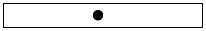'()(make-node '() '())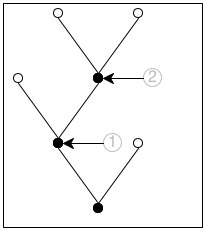(make-node (make-node '() (make-node '() '())) '())

Figure 184: Some stripped-down binary trees

To transform this function into an accumulator-style function, we follow the standard path. We begin with an appropriate template:
 (define (height.v2 abt0) (local (; Tree ??? -> N ; measures the height of abt ; accumulator ... (define (height/a abt a) (cond [(empty? abt) ...] [else (... (height/a (node-left abt) ) ... ... (height/a (node-right abt) ) ...)]))) (height/a abt0 ...)))
As always, the problem is to determine what knowledge the accumulator represents. One obvious choice is the number of traversed branches:

a is the number of steps it takes to reach abt from abt0.

Illustrating this accumulator invariant is best done with a graphical example. Take a second look at figure 184. The bottom-most tree comes with two annotations, each pointing out one subtree:
1. If abt0 is the complete tree and abt is the subtree pointed to by the circled 1, the accumulator’s value must be 1 because it takes exactly one step to get from the root of abt to the root of abt0.

2. In the same spirit, for the subtree labeled 2 the accumulator is 2 because it takes two steps to get to this place.

As for the preceding two examples, the invariant basically dictates how to follow the rest of the design recipe for accumulators: the initial value for a is 0; the update operation is add1; and the base case uses the accumulated knowledge by returning it. Translating this into code yields the following skeleton definition:
 (define (height.v2 abt0) (local (; Tree N -> N ; measures the height of abt ; accumulator a is the number of steps ; it takes to reach abt from abt0 (define (height/a abt a) (cond [(empty? abt) a] [else (... (height/a (node-left abt) (+ a 1)) ... ... (height/a (node-right abt) (+ a 1)) ...)]))) (height/a abt0 0)))
But, in contrast to the first two examples, a is not the final result. In the second cond clause, the two recursive calls yield two values. The design recipe for structural functions dictates that we combine those in order to formulate an answer for this case; the dots above indicate that we still need to pick an operation that combines these values.

 ; Tree -> N ; measures the height of abt0 (check-expect (height.v2 example) 3) (define (height.v2 abt0) (local (; Tree N -> N ; measures the height of abt ; accumulator a is the number of steps ; it takes to reach abt from abt0 (define (height/a abt a) (cond [(empty? abt) a] [else (max (height/a (node-left abt)  (+ a 1)) (height/a (node-right abt) (+ a 1)))]))) (height/a abt0 0)))

Figure 185: The accumulator-style version of height

Following the design recipe also tells us that we need to interpret the two values to find the appropriate function. According to the purpose statement for height/a, the first value is the height of the left subtree, and the second one is the height of the right one. Given that we are interested in the height of abt itself and that the height is the largest number of steps it takes to reach a leaf, we use the max function to pick the proper one; see figure 185 for the complete definition.

Note on an Alternative Design In addition to counting the number of steps it takes to reach a node, an accumulator function could hold on to the largest height encountered so far. Here is the accumulator statement for the design idea:

The first accumulator, a, represents the number of steps it takes to reach abt from abt0, and the second accumulator stands for the tallest branch in the part of abt0 that is to the left of abt.

Clearly, this statement assumes a template with two accumulator parameters, something we have not encountered before:
 ... ; Tree N N -> N ; measures the height of abt ; accumulator s is the number of steps ; it takes to reach abt from abt0 ; accumulator m is the maximal height of ; the part of abt0 that is to the left of abt (define (h/a abt s m) (cond [(empty? abt) ...] [else (... (h/a (node-left abt) ) ... ... (h/a (node-right abt) ) ...)])) ...

Exercise 498. Complete the design of height.v3. Hint In terms of the bottom-most tree of figure 184, the place marked 1 has no complete paths to leafs to its left while the place marked 2 has one complete path and it consists of two steps.

This second design has a more complex accumulator invariant than the first one. By implication, its implementation requires more care than the first one. At the same time, it comes without any advantages, meaning it is inferior to the first one.

Our point is that different accumulator invariants yield different variants. You can design both variants systematically, following the same design recipe. When you have complete function definitions, you can compare and contrast the results, and you can then decide which one to keep, based on evidence. End

Exercise 499. Design an accumulator-style version of product, the function that computes the product of a list of numbers. Stop when you have formulated the accumulator invariant and have someone check it.

The performance of product is O(n) where n is the length of the list. Does the accumulator version improve on this?

Exercise 500. Design an accumulator-style version of how-many, which is the function that determines the number of items on a list. Stop when you have formulated the invariant and have someone check it.

The performance of how-many is O(n) where n is the length of the list. Does the accumulator version improve on this?

Computer scientists refer to this space as stack space, but you can safely ignore this terminology for now.

When you evaluate (how-many some-non-empty-list) by hand, n applications of add1 are pending by the time the function reaches '()where n is the number of items on the list. Computer scientists sometime say that how-many needs O(n) space to represent these pending function applications. Does the accumulator reduce the amount of space needed to compute the result?

Exercise 501. Design an accumulator-style version of add-to-pi. The function adds a natural number to pi without using +:
 ; N -> Number ; adds n to pi without using + (check-within (add-to-pi 2) (+ 2 pi) 0.001) (define (add-to-pi n) (cond [(zero? n) pi] [else (add1 (add-to-pi (sub1 n)))]))
Stop when you have formulated the accumulator invariant and have someone check it.

Exercise 502. Design the function palindrome, which accepts a non-empty list and constructs a palindrome by mirroring the list around the last item. When given (explode "abc"), it yields (explode "abcba").

Hint Here is a solution designed by function composition:
 ; [NEList-of 1String] -> [NEList-of 1String] ; creates a palindrome from s0 (check-expect (mirror (explode "abc")) (explode "abcba")) (define (mirror s0) (append (all-but-last s0) (list (last s0)) (reverse (all-but-last s0))))
See Auxiliary Functions that Generalize for last; design all-but-last in an analogous manner.

This solution traverses s0 four times:
1. via all-but-last,

2. via last,

3. via all-but-last again, and

4. via reverse, which is ISL+’s version of invert.

Even with local definition for the result of all-but-last, the function needs three traversals. While these traversals aren’t “stacked” and therefore don’t have a disastrous impact on the function’s performance, an accumulator version can compute the same result with a single traversal.

Exercise 503. Exercise 467 implicitly asks for the design of a function that rotates a Matrix until the first coefficient of the first row differs from 0. In the context of Exercise 467, the solution calls for a generative-recursive function that creates a new matrix by shifting the first row to the end when it encounters a 0 in the first position. Here is the solution:
 ; Matrix -> Matrix ; finds a row that doesn't start with 0 and ; uses it as the first one ; generative moves the first row to last place ; no termination if all rows start with 0 (check-expect (rotate-until.v2 '((0 4 5) (1 2 3))) '((1 2 3) (0 4 5))) (define (rotate M) (cond [(not (= (first (first M)) 0)) M] [else (rotate (append (rest M) (list (first M))))]))
Stop! Modify this function so that it signals an error when all rows start with 0.

If you measure this function on large instances of Matrix, you get a surprising result:
 rows in M 1000 2000 3000 4000 5000 rotate 17 66 151 272 436
As the number of rows increases from 1,000 to 5,000, the time spent by rotate does not increase by a factor of five but by twenty.

The problem is that rotate uses append, which makes a brand-new list like (rest M) only to add (first M) at the end. If M consists of 1,000 rows and the last row is the only one with a non-0 coefficient, that’s roughly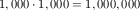lists. How many lists do we get if M consists of 5,000 lines?

Now suppose we conjecture that the accumulator-style version is faster than the generative one. Here is the accumulator template for a structurally recursive version of rotate:
 (define (rotate.v2 M0) (local (; Matrix ... -> Matrix ; accumulator ... (define (rotate/a M seen) (cond [(empty? M) ...] [else (... (rotate/a (rest M) ... seen ...) ...)]))) (rotate/a M0 ...)))
The goal is to remember the first row when its leading coefficient is 0 without using append for every recursion.

Formulate an accumulator statement. Then follow the accumulator design recipe to complete the above function. Measure how fast it runs on a Matrix that consists of rows with leading 0s except for the last one. If you completed the design correctly, the function is quite fast.

Exercise 504. Design to10. It consumes a list of digits and produces the corresponding number. The first item on the list is the most significant digit. Hence, when applied to '(1 0 2), it produces 102.

Domain Knowledge You may recall from grade school that the result is determined byExercise 505. Design the function is-prime, which consumes a natural number and returns #true if it is prime and #false otherwise.

Domain Knowledge A number n is prime if it is not divisible by any number between n - 1 and 2.

Hint The design recipe for N [>=1] suggests the following template:
 ; N [>=1] -> Boolean ; determines whether n is a prime number (define (is-prime? n) (cond [(= n 1) ...] [else (... (is-prime? (sub1 n)) ...)]))
This template immediately tells you that the function forgets n, its initial argument as it recurs. Since n is definitely needed to determine whether n is divisible by (- n 1), (- n 2), and so on, you know that you need an accumulator-style function.

Note on Speed Programmers who encounter accumulator-style functions for the first time often get the impression that they are always faster than their plain counterparts. So let’s take a look at the solution of exercise 497:An explanation of these times is beyond the scope of this book.
 !.v1 5.76 5.78 5.8 5.82 5.87 5.806 !.v2 5.97 5.94 5.98 5.97 6.69 6.111
The table’s top row shows the number of seconds for five runs of (!.v1 20), while the bottom one lists those of running (!.v2 20). The last column shows the averages. In short, the table shows that people jump to premature conclusions; the performance of at least one accumulator-style function is worse than that of the original. Do not trust prejudices. Instead, measure performance characteristics of your programs for yourself. End

Exercise 506. Design an accumulator-style version of map.

Exercise 507. Exercise 257 explains how to design foldl with the design recipes and guidelines of the first two parts of the book:
 (check-expect (f*ldl + 0 '(1 2 3)) (foldl + 0 '(1 2 3))) (check-expect (f*ldl cons '() '(a b c)) (foldl cons '() '(a b c))) ; version 1 (define (f*ldl f e l) (foldr f e (reverse l)))
That is, foldl is the result of reversing the given list and then using foldr to fold the given function over this intermediate list.

The f*ldl function obviously traverses the list twice, but once we design all the functions, it becomes clear how much harder it has to work:
 ; version 2 (define (f*ldl f e l) (local ((define (reverse l) (cond [(empty? l) '()] [else (add-to-end (first l) (reverse (rest l)))])) (define (add-to-end x l) (cond [(empty? l) (list x)] [else (cons (first l) (add-to-end x (rest l)))])) (define (foldr l) (cond [(empty? l) e] [else (f (first l) (foldr (rest l)))]))) (foldr (reverse l))))
We know that reverse has to traverse a list once for every item on the list, meaning f*ldl really performstraversals for a list of length n. Fortunately, we know how to eliminate this bottleneck with an accumulator:
 ; version 3 (define (f*ldl f e l) (local ((define (invert/a l a) (cond [(empty? l) a] [else (invert/a (rest l) (cons (first l) a))])) (define (foldr l) (cond [(empty? l) e] [else (f (first l) (foldr (rest l)))]))) (foldr (invert/a l '()))))

Once reverse uses an accumulator, we actually get the apparent performance of two traversals of the list. The question is whether we can improve on this by adding an accumulator to the locally defined fold:
 ; version 4 (define (f*ldl f e l0) (local ((define (fold/a a l) (cond [(empty? l) a] [else (fold/a (f (first l) a) (rest l))]))) (fold/a e l0)))
Since equipping the function with an accumulator reverses the order in which the list is traversed, the initial reversal of the list is superfluous.

Task 1 Recall the signature for foldl:

; [X Y] [X Y -> Y] Y [List-of X] -> Y

It is also the signature of f*ldl. Formulate the signature for fold/a and its accumulator invariant. Hint Assume that the difference between l0 and l is (list x1 x2 x3). What is a, then?

You may also be wondering why fold/a consumes its arguments in this unusual order, first the accumulator and then the list. To understand the reason for this ordering, imagine instead that fold/a also consumes fas the first argument. At this point it becomes abundantly clear that fold/a is foldl:
 ; version 5 (define (f*ldl f i l) (cond [(empty? l) i] [else (f*ldl f (f (first l) i) (rest l))]))

Task 2 Design build-l*st using an accumulator-style approach. The function must satisfy the following tests:

(check-expect (build-l*st n f) (build-list n f))

for any natural number n and function f.

#### 32.4A Graphical Editor, with Mouse

A Graphical Editor introduces the notion of a one-line editor and presents a number of exercises on creating a graphical editor. Recall that a graphical editor is an interactive program that interprets key events as editing actions on a string. In particular, when a user presses the left or right arrow keys, the cursor moves left or right; similarly, pressing the delete key removes a 1String from the edited text. The editor program uses a data representation that combines two strings in a structure. A Graphical Editor, Revisited resumes these exercises and shows how the same program can greatly benefit from a different data structure, one that combines two strings.

Neither of these sections deals with mouse actions for navigation, even though all modern applications support this functionality. The basic difficulty with mouse events is to place the cursor at the appropriate spot. Since the program deals with a single line of text, a mouse click at (x,y) clearly aims to place the cursor between the letters that are visible at or around the x position. This section fills the gap.

Recall the relevant definitions from A Graphical Editor, Revisited:

 (define FONT-SIZE 11) (define FONT-COLOR "black") ; [List-of 1String] -> Image ; renders a string as an image for the editor (define (editor-text s) (text (implode s) FONT-SIZE FONT-COLOR)) (define-struct editor [pre post]) ; An Editor is a structure: ;   (make-editor [List-of 1String] [List-of 1String]) ; interpretation if (make-editor p s) is the state of ; an interactive editor, (reverse p) corresponds to ; the text to the left of the cursor and s to the ; text on the right

Exercise 508. Design split-structural using the structural design recipe. The function consumes a list of 1Strings ed and a natural number x; the former represents the complete string in some Editor and the latter the x-coordinate of the mouse click. The function produces

(make-editor p s)

such that (1) p and s make up ed and (2) x is larger than the image of p and smaller than the image of p extended with the first 1String on s (if any).

Here is the first condition expressed with an ISL+ expression:

(string=? (string-append p s) ed)

The second one is
 (<= (image-width (editor-text p)) x (image-width (editor-text (append p (first s)))))
assuming (cons? s).

Hints (1) The x-coordinate measures the distance from the left. Hence the function must check whether smaller and smaller prefixes of ed fit into the given width. The first one that doesn’t fit corresponds to the pre field of the desired Editor, the remainder of ed to the post field.

(2) Designing this function calls for thoroughly developing examples and tests. See Intervals, Enumerations, and Itemizations.

Exercise 509. Design the function split. Use the accumulator design recipe to improve on the result of exercise 508. After all, the hints already point out that when the function discovers the correct split point, it needs both parts of the list, and one part is obviously lost due to recursion.

Once you have solved this exercise, equip the main function of A Graphical Editor, Revisited with a clause for mouse clicks. As you experiment with moving the cursor via mouse clicks, you will notice that it does not exactly behave like applications that you use on your other devices—even though split passes all its tests.

Graphical programs, like editors, call for experimentation to come up with the best “look and feel” experiences. In this case, your editor is too simplistic with its placement of the cursor. After the applications on your computer determine the split point, they also determine which letter division is closer to the x-coordinate and place the cursor there.

Exercise 510. Many operating systems come with the fmt program, which can rearrange the words in a file so that all lines in the resulting file have a maximal width. As a widely used program, fmt supports a range of related functions. This exercise focuses on its core functionality.

Design the program fmt. It consumes a natural number w, the name of an input file in-f, and the name of an output file out-fin the same sense as read-file from the 2htdp/batch-io library. Its purpose is to read all the words from the in-f, to arrange these words in the given order into lines of maximal width w, and to write these lines to out-f.

### 33More Uses of Accumulation

This chapter presents three more uses of accumulators. The first section concerns the use of accumulators in conjunction with tree-processing functions. It uses the compilation of ISL+ as an illustrative example. The second section explains why we occasionally want accumulators inside of data representations and how to go about placing them there. The final section resumes the discussion of rendering fractals.

#### 33.1Accumulators and Trees

When you ask DrRacket to run an ISL+ program, it translates the program to commands for your specific computer. This process is called compilation, and the part of DrRacket that performs the task is called a compiler. Before the compiler translates the ISL+ program, it checks that every variable is declared via a define, a define-struct, or a lambda.

Stop! Enter x, (lambda (y) x), and (x 5) as complete ISL+ programs into DrRacket and ask it to run each. What do you expect to see?

Let’s phrase this idea as a sample problem:

Sample Problem You have been hired to re-create a part of the ISL+ compiler. Specifically, your task deals with the following language fragment, specified in the so-called grammar notation that many programming language manuals use:We use the Greek letter λ instead of lambda to signal that this exercise deals with ISL+ as an object of study, not just a programming language.
 expression = variable | (λ (variable) expression) | (expression expression)
Remember from intermezzo 1 that you can read the grammar aloud replacing = with “is one of” and | with “or.”

Recall that λ expressions are functions without names. They bind their parameter in their body. Conversely, a variable occurrence is declared by a surrounding λ that specifies the same name as a parameter. You may wish to revisit Intermezzo 3: Scope and Abstraction because it deals with the same issue from the perspective of a programmer. Look for the terms “binding occurrence,” “bound occurrence,” and “free.”

Develop a data representation for the above language fragment; use symbols to represent variables. Then design a function that replaces all undeclared variables with '*undeclared.

This problem is representative of many steps in the translation process and, at the same time, is a great case study for accumulator-style functions.

Before we dive into the problem, let’s look at some examples in this mini-language, recalling what we know about lambda:
• (λ (x) x) is the function that returns whatever it is given, also known as the identity function;

• (λ (x) y) looks like a function that returns y whenever it is given an argument, except that y isn’t declared;

• (λ (y) (λ (x) y)) is a function that, when given some value v, produces a function that always returns v;

• ((λ (x) x) (λ (x) x)) applies the identity function to itself;

• ((λ (x) (x x)) (λ (x) (x x))) is a short infinite loop; and

• (((λ (y) (λ (x) y)) (λ (z) z)) (λ (w) w)) is a complex expression that is best run in ISL+ to find out whether it terminates.

Indeed, you can run all of the above ISL+ expressions in DrRacket to confirm what is written about them.

Exercise 511. Explain the scope of each binding occurrence in the above examples. Draw arrows from the bound to the binding occurrences.

Developing a data representation for the language is easy, especially because its description uses a grammar notation. Here is one possibility:
 ; A Lam is one of: ; – a Symbol ; – (list 'λ (list Symbol) Lam) ; – (list Lam Lam)
Because of quote, this data representation makes it easy to create data representations for expressions in our subset of ISL+:
 (define ex1 '(λ (x) x)) (define ex2 '(λ (x) y)) (define ex3 '(λ (y) (λ (x) y))) (define ex4 '((λ (x) (x x)) (λ (x) (x x))))
These four data examples are representations of some of the above expressions. Stop! Create data representations for the remaining examples.

Exercise 512. Define is-var?, is-λ?, and is-app?, that is, predicates that distinguish variables from λ expressions and applications.

Also define
• λ-para, which extracts the parameter from a λ expression;

• λ-body, which extracts the body from a λ expression;

• app-fun, which extracts the function from an application; and

• app-arg, which extracts the argument from an application.

With these predicates and selectors, you basically can act as if you had defined a structure-oriented data representation.

Design declareds, which produces the list of all symbols used as λ parameters in a λ term. Don’t worry about duplicate symbols.

Exercise 513. Develop a data representation for the same subset of ISL+ that uses structures instead of lists. Also provide data representations for ex1, ex2, and ex3 following your data definition.

We follow the structural design recipe, and here is the product of steps two and three:
 ; Lam -> Lam ; replaces all symbols s in le with '*undeclared ; if they do not occur within the body of a λ ; expression whose parameter is s (check-expect (undeclareds ex1) ex1) (check-expect (undeclareds ex2) '(λ (x) *undeclared)) (check-expect (undeclareds ex3) ex3) (check-expect (undeclareds ex4) ex4) (define (undeclareds le0) le0)
Note how we expect undeclareds to process ex4 even though the expression loops forever when run; compilers don’t run programs, they read them and create others.

A close look at the purpose statement directly suggests that the function needs an accumulator. This becomes even clearer when we inspect the template for undeclareds:
 (define (undeclareds le) (cond [(is-var? le) ...] [(is-λ? le) (... (undeclareds (λ-body le)) ...)] [(is-app? le) (... (undeclareds (app-fun le)) ... (undeclareds (app-arg le)) ...)]))
When undeclareds recurs on the body of (the representation of) a λ expression, it forgets (λ-para le), the declared variable.

 (define (undeclareds le0) (local (; Lam ??? -> Lam ; accumulator a represents ... (define (undeclareds/a le a) (cond [(is-var? le) ...] [(is-λ? le) (... (undeclareds/a (λ-body le) ... a ...) ...)] [(is-app? le) (... (undeclareds/a (app-fun le) ... a ...) ... (undeclareds/a (app-arg le) ... a ...) ...)]))) (undeclareds/a le0 ...)))
In this context, we can now formulate an accumulator invariant:

a represents the list of λ parameters encountered on the path from the top of le0 to the top of le.

For example, if le0 is

'(((λ (y) (λ (x) y)) (λ (z) z)) (λ (w) w))

and le is the highlighted subtree, then a contains y. The left side of figure 186 presents a graphical illustration of the same example. It shows a Lam expression as an upside-down tree; that is, the root is at the top. A @ node represents an application with two descendants; the other nodes are self-explanatory. In this tree diagram, the bold path leads from le0 to le through a single variable declaration.

Similarly, if we pick a different subtree of the same piece of data,

'(((λ (y) (λ (x) y)) (λ (z) z)) (λ (w) w))

we get an accumulator that contains both 'y and 'x. The right side of figure 186 makes this point again. Here the bold path leads through two 'λ nodes to the boxed subtree, and the accumulator is the list of declared variables along the bold path.Figure 186: Lam terms as trees

Now that we have settled on the data representation of the accumulator and its invariant, we can resolve the remaining design questions:
• We pick an initial accumulator value of '().

• We use cons to add (λ-para le) to a.

• We exploit the accumulator for the clause where undeclareds/a deals with a variable. Specifically, the function uses the accumulator to check whether the variable is in the scope of a declaration.

Figure 187 shows how to translate these ideas into a complete function definition. Note the name declareds for the accumulator; it brings across the key idea behind the accumulator invariant, helping the programmer understand the definition. The base case uses member? from ISL+ to determine whether the variable le is in declareds and, if not, replaces it with '*undeclared. The second cond clause uses a local to introduce the extended accumulator newd. Because para is also used to rebuild the expression, it has its own local definition. Finally, the last clause concerns function applications, which do not declare variables and do not use any directly. As a result, it is by far the simplest of the three clauses.

 ; Lam -> Lam (define (undeclareds le0) (local (; Lam [List-of Symbol] -> Lam ; accumulator declareds is a list of all λ ; parameters on the path from le0 to le (define (undeclareds/a le declareds) (cond [(is-var? le) (if (member? le declareds) le '*undeclared)] [(is-λ? le) (local ((define para (λ-para le)) (define body (λ-body le)) (define newd (cons para declareds))) (list 'λ (list para) (undeclareds/a body newd)))] [(is-app? le) (local ((define fun (app-fun le)) (define arg (app-arg le))) (list (undeclareds/a fun declareds) (undeclareds/a arg declareds)))]))) (undeclareds/a le0 '())))

Figure 187: Finding undeclared variables

Exercise 514. Make up an ISL+ expression in which x occurs both free and bound. Formulate it as an element of Lam. Does undeclareds work properly on your expression?

Exercise 515. Consider the following expression:

(λ (*undeclared) ((λ (x) (x *undeclared)) y))

Yes, it uses *undeclared as a variable. Represent it in Lam and check what undeclareds produces for this expression.

Modify undeclareds so that it replaces a free occurrence of 'x with

(list '*undeclared 'x)

and a bound one 'y with

(list '*declared 'y)

Doing so unambiguously identifies problem spots, which a program development environment such as DrRacket can use to highlight errors.

Note The trick of replacing a variable occurrence with the representation of an application feels awkward. If you dislike it, consider synthesizing the symbols '*undeclared:x and 'declared:y instead.

Exercise 516. Redesign the undeclareds function for the structure-based data representation from exercise 513.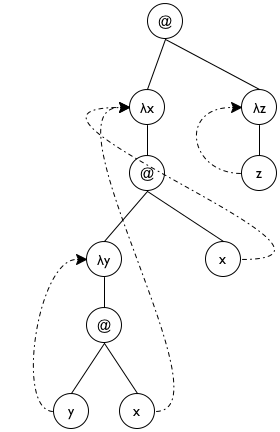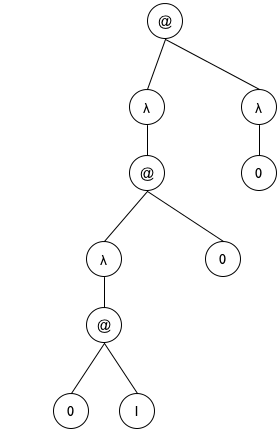Figure 188: Static distances

Exercise 517. Design static-distance. The function replaces all occurrences of variables with a natural number that represents how far away the declaring λ is. Figure 188 illustrates the idea for the term

'((λ (x) ((λ (y) (y x)) x)) (λ (z) z))

in graphical form. It includes dotted arrows that point from variable occurrences to the corresponding variable declarations. On the right, the figure shows a tree of the same shape, though without the arrows. The 'λ nodes come without names, and variable occurrences have been replaced by natural numbers that specify which 'λ declares the variable. Each natural number n says that the binding occurrence is n steps upward—toward the root of the Lam tree. A value of 0 denotes the first 'λ on the path to the root, 1 the second one, and so on.

Hint The undeclareds accumulator of undeclareds/a is a list of all parameters on path from le to le0 in reverse orderthe last one seen is first on the list.

#### 33.2Data Representations with Accumulators

The end of Intermezzo 5: The Cost of Computation explains that *SL measures the size of containers, say lists, by traversing them and hints that other programming languages use a different,See Finite State Machines for an early example of this idea. less expensive way, to compute sizes. In this section, we show how to implement this idea with the addition of an accumulator to data representations.

Consider the ubiquitous lists in *SL. All lists are constructed from cons and '(); operations such as quote and list, for example, are merely abbreviations for these two. As What Is '(), What Is cons shows, it is also possible to mimic lists in BSL with suitable structure type and function definitions.

 (define-struct pair [left right]) ; ConsOrEmpty is one of: ; – '() ; – (make-pair Any ConsOrEmpty) ; Any ConsOrEmpty -> ConsOrEmpty (define (our-cons a-value a-list) (cond [(empty? a-list) (make-pair a-value a-list)] [(our-cons? a-list) (make-pair a-value a-list)] [else (error "our-cons: ...")])) ; ConsOrEmpty -> Any ; extracts the left part of the given pair (define (our-first mimicked-list) (if (empty? mimicked-list) (error "our-first: ...") (pair-left mimicked-list)))

Figure 189: An implementation of lists in BSL

Figure 189 recalls the basic idea. Stop! Can you define our-rest now?

The key insight is that we can add a third field to the structure type definition of pair:
 (define-struct cpair [count left right]) ; A [MyList X] is one of: ; – '() ; – (make-cpair (tech "N") X [MyList X]) ; accumulator the count field is the number of cpairs
As the accumulator statement says, the extra field is used to keep track of the number of cpair instances used to create the list. That is, it remembers a fact about the construction of the list. We call this kind of structure field a data accumulator.

Adding a field to the major list constructor does not come for free. To begin with, it requires a change to the checked version of the constructor, the one that is actually available to programs:
 ; data definitions, via a constructor-function (define (our-cons f r) (cond [(empty? r) (make-cpair 1 f r)] [(cpair? r) (make-cpair (+ (cpair-count r) 1) f r)] [else (error "our-cons: ...")]))
If the extended list is '(), count is populated with 1; otherwise, the function computes the length from the given cpair.

Now the function definition for our-length is obvious:
 ; Any -> N ; how many items does l contain (define (our-length l) (cond [(empty? l) 0] [(cpair? l) (cpair-count l)] [else (error "my-length: ...")]))
The function consumes any kind of value. For '() and instances of cpair, it produces natural numbers; otherwise it signals an error.

The second problem with the addition of a count field concerns performance. Indeed, there are two concerns. On the one hand, every single list construction comes with an extra field now, meaning a 33% increase in memory consumption. On the other hand, the addition of the field decreases how fast our-cons constructs a list. In addition to the check that the extended list is either '() or an instance of a cpair, the constructor now computes the size of the list. Although this computation consumes a constant amount of time, it is imposed on every single use of our-consand just think how many times this book uses cons and does not ever compute how long the resulting list is!

Exercise 518. Argue that our-cons takes a constant amount of time to compute its result, regardless of the size of its input.

Exercise 519. Is it acceptable to impose the extra cost on cons for all programs to turn length into a constant-time function?

While the addition of a count field to lists is questionable, sometimes data accumulators play a crucial role in finding a solution. The next example is about adding so-called artificial intelligence to a board-game-playing program, and its data accumulator is an absolute necessity.

As you play board games or solve puzzles, you tend to think about your possible moves at every stage. As you get better, you may even imagine the possibilities after this first step. The result is a so-called game tree, which is a (part of the) tree of all possible moves that the rules allow. Let’s start with a problem:

Sample Problem Your manager tells you the following story.

“Once upon a time, three cannibals were guiding three missionaries through a jungle. They were on their way to the nearest mission station. After some time, they arrived at a wide river, filled with deadly snakes and fish. There was no way to cross the river without a boat. Fortunately, they found a rowboat with two oars after a short search. Unfortunately, the boat was too small to carry all of them. It could barely carry two people at a time. Worse, because of the river’s width someone had to row the boat back.

“Since the missionaries could not trust the cannibals, they had to figure out a plan to get all six of them safely across the river. The problem was that these cannibals would kill and eat missionaries as soon as there were more cannibals than missionaries in some place. Our missionaries had to devise a plan that guaranteed that there were never any missionaries in the minority on either side of the river. The cannibals, however, could be trusted to cooperate otherwise. Specifically, they would not abandon any potential food, just as the missionaries would not abandon any potential converts.”

While your manager doesn’t assign any specific design task, he wants to explore whether the company can design (and sell) programs that solve such puzzles.

While puzzles aren’t board games, the program illustrates the idea of game trees in the most straightforward manner possible.

In principle, it is quite straightforward to solve such puzzles by hand. Here is the rough idea. Pick a graphical representation of the problem states. Ours consists of a three-part box: the left one represents the missionaries and the cannibals; the middle combines the river and the boat; and the third part is the right-hand side of the river. Take a look at the following representation of the initial state: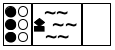Black circles denote missionaries, white circles cannibals. All of them are on the left-hand river bank. The boat is also on the left side. Nobody is on the right. Here are two more states: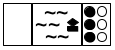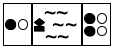The first one is the final state, where all people and the boat are on the right bank of the river. The second one depicts some intermediate state where two people are on the left with the boat and four people are on the right.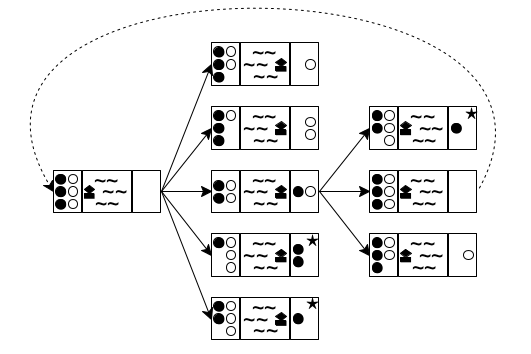Figure 190: Creating a game tree

Now that you have a way to write down the state of the puzzle, you can think about the possibilities at each stage. Doing so yields a tree of possible moves. Figure 190 sketches the first two and a half layers in such a tree. The left-most state is the initial one. Because the boat can transport at most two people and must be rowed by at least one, you have five possibilities to explore: one cannibal rows across; two cannibals row across; one missionary and one cannibal go; one missionary crosses; or two missionaries do. These possibilities are represented with five arrows going from the initial state to five intermediate states.

For each of these five intermediate states, you can play the same game again. In figure 190 you see how the game continues for the middle (third) one of the new states. Because there are only two people on the right river bank, you see three possibilities: a cannibal goes back, a missionary goes back, or both do. Hence three arrows connect the middle state to the three states on the right side of the tree. If you keep drawing this tree of possibilities in a systematic manner, you eventually discover the final state.

A second look at figure 190 reveals two problems with this naive approach to generating the tree of possibilities. The first one is the dashed arrow that connects the middle state on the right to the initial state. It indicates that rowing back the two people from the right to the left gets the puzzle back to its initial state, meaning you’re starting over, which is obviously undesirable. The second problem concerns those states with a star in the top-right corner. In both cases, there are more white-circle cannibals than black-circle missionaries on the left river bank, meaning the cannibals would eat the missionaries. Again, the goal is to avoid such states, making these moves undesirable.

One way to turn this puzzle into a program is to design a function that determines whether some final state—here the final state—is reachable from some given state. Here is an appropriate function definition:
 ; PuzzleState -> PuzzleState ; is the final state reachable from state0 ; generative creates a tree of possible boat rides ; termination ??? (check-expect (solve initial-puzzle) final-puzzle) (define (solve state0) (local (; [List-of PuzzleState] -> PuzzleState ; generative generates the successors of los (define (solve* los) (cond [(ormap final? los) (first (filter final? los))] [else (solve* (create-next-states los))]))) (solve* (list state0))))
The auxiliary function uses generative recursion, generating all new possibilities given a list of possibilities. If one of the given possibilities is a final state, the function returns it.

Clearly, solve is quite generic. As long as you define a collection of PuzzleStates, a function for recognizing final states, and a function for creating all “successor” states, solve can work on your puzzle.

Exercise 520. The solve* function generates all states reachable with n boat trips before it looks at states that require n + 1 boat trips, even if some of those boat trips return to previously encountered states. Because of this systematic way of traversing the tree, solve* cannot go into an infinite loop. Why? Terminology This way of searching a tree or a graph is dubbed breadth-first search.

Exercise 521. Develop a representation for the states of the missionary-and-cannibal puzzle. Like the graphical representation, a data representation must record the number of missionaries and cannibals on each side of the river plus the location of the boat.

The description of PuzzleState calls for a new structure type. Represent the above initial, intermediate, and final states in your representation.

Design the function final?, which detects whether in a given state all people are on the right river bank.

Design the function render-mc, which maps a state of the missionary-and-cannibal puzzle to an image.

The problem is that returning the final state says nothing about how the player can get from the initial state to the final one. In other words, create-next-states forgets how it gets to the returned states from the given ones. And this situation clearly calls for an accumulator, but at the same time, the accumulated knowledge is best associated with every individual PuzzleState, not solve* or any other function.

Exercise 522. Modify the representation from exercise 521 so that a state records the sequence of states traversed to get there. Use a list of states.

Articulate and write down an accumulator statement with the data definition that explains the additional field.

Modify final? or render-mc for this representation as needed.

Exercise 523. Design the create-next-states function. It consumes lists of missionary-and-cannibal states and generates the list of all those states that a boat ride can reach.

Ignore the accumulator in the first draft of create-next-states, but make sure that the function does not generate states where the cannibals can eat the missionaries.

For the second design, update the accumulator field in the state structures and use it to rule out states that have been encountered on the way to the current state.

Exercise 524. Exploit the accumulator-oriented data representation to modify solve. The revised function produces the list of states that lead from the initial PuzzleState to the final one.

Also consider creating a movie from this list, using render-mc to generate the images. Use run-movie to display the movie.

#### 33.3Accumulators as Results

Take another look at figure 157. It displays a Sierpinski triangle and a suggestion how to create it. Specifically, the images on the right explain one version of the generative idea behind the process:

The given problem is a triangle. When the triangle is too small to be subdivided any further, the algorithm does nothing; otherwise, it finds the midpoints of its three sides and deals with the three outer triangles recursively.

In contrast, Fractals, a First Taste shows how to compose Sierpinski triangles algebraically, a process that does not correspond to this description.

Most programmers expect “draw” to mean the action of adding a triangle to some canvas. The scene+line function from the 2htdp/image library makes this idea concrete. The function consumes an image s and the coordinates of two points and adds a line through these two points to s. It is easy to generalize from scene+line to add-triangle and from there to add-sierpinski:

Sample Problem Design the add-sierpinski function. It consumes an image and three Posns describing a triangle. It uses the latter to add a Sierpinski triangle to this image.

Note how this problem implicitly refers to the above process description of how to draw a Sierpinski triangle. In other words, we are confronted with a classical generative-recursive problem, and we can start with the classic template of generative recursion and the four central design questions:
• The problem is trivial if the triangle is too small to be subdivided.

• In the trivial case, the function returns the given image.

• Otherwise the midpoints of the sides of the given triangle are determined to add another triangle. Each “outer” triangle is then processed recursively.

• Each of these recursive steps produces an image. The remaining question is how to combine these images.

 ; Image Posn Posn Posn -> Image ; generative adds the triangle (a, b, c) to s, ; subdivides it into three triangles by taking the ; midpoints of its sides; stop if (a, b, c) is too small (define (add-sierpinski scene0 a b c) (cond [(too-small? a b c) scene0] [else (local ((define scene1 (add-triangle scene0 a b c)) (define mid-a-b (mid-point a b)) (define mid-b-c (mid-point b c)) (define mid-c-a (mid-point c a)) (define scene2 (add-sierpinski scene0 a mid-a-b mid-c-a)) (define scene3 (add-sierpinski scene0 b mid-b-c mid-a-b)) (define scene4 (add-sierpinski scene0 c mid-c-a mid-b-c))) ; —IN— (... scene1 ... scene2 ... scene3 ...))]))

Figure 191: Accumulators as results of generative recursions, a skeleton

Figure 191 shows the result of translating these answers into a skeletal definition. Since each midpoint is used twice, the skeleton uses local to formulate the generative step in ISL+. The local expression introduces the three new midpoints plus three recursive applications of add-sierpinski. The dots in its body suggest a combination of the scenes.

Exercise 525. Tackle the wish list that the skeleton implies:
 ; Image Posn Posn Posn -> Image ; adds the black triangle a, b, c to scene (define (add-triangle scene a b c) scene) ; Posn Posn Posn -> Boolean ; is the triangle a, b, c too small to be divided (define (too-small? a b c) #false) ; Posn Posn -> Posn ; determines the midpoint between a and b (define (mid-point a b) a)
Design the three functions.

Domain Knowledge (1) For the too-small? function it suffices to measure the distance between two points and to check whether it is below some chosen threshold, say, 10. The distance between (x0,y0) and (x1,y1) is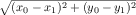that is, the distance of (x0 - x1,y0 - y1) to the origin.

The midpoint between points (x0,y0) and (x1,y1) has as coordinates the midpoints between the respective x and y coordinates: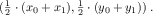Now that we have all the auxiliary functions, it is time to return to the problem of combining the three images that are created by the recursive calls. One obvious guess is to use the overlay or underlay function, but an evaluation in the interactions area of DrRacket shows that the functions hide the underlying triangles.

Specifically, imagine that the three recursive calls produce the following empty scenes, enriched with a single triangle in appropriate locations:
 > scene1> scene2> scene3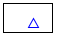A combination should look like this figure:But, combining these shapes with overlay or underlay does not yield this desired shape:
 > (overlay scene1 scene2 scene3)> (underlay scene1 scene2 scene3)Indeed, the image library of ISL+ does not support a function that combines these scenes in an appropriate manner.

Let’s take a second look at these interactions. If scene1 is the result of adding the upper triangle to the given scene and scene2 is the result of adding a triangle on the lower left, perhaps the second recursive call should add triangles to the result of the first call. Doing so would yield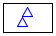and handing over this scene to the third recursive call produces exactly what is wanted: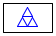; Image Posn Posn Posn -> Image ; generative adds the triangle (a, b, c) to s, ; subdivides it into three triangles by taking the ; midpoints of its sides; stop if (a, b, c) is too small ; accumulator the function accumulates the triangles scene0 (define (add-sierpinski scene0 a b c) (cond [(too-small? a b c) scene0] [else (local ((define scene1 (add-triangle scene0 a b c)) (define mid-a-b (mid-point a b)) (define mid-b-c (mid-point b c)) (define mid-c-a (mid-point c a)) (define scene2 (add-sierpinski scene1 a mid-a-b mid-c-a)) (define scene3 (add-sierpinski scene2 b mid-b-c mid-a-b))) ; —IN— (add-sierpinski scene3 c mid-c-a mid-b-c))]))

Figure 192: Accumulators as results of generative recursion, the function

Figure 192 shows the reformulation based on this insight. The three highlights pinpoint the key design idea. All concern the case when the triangle is sufficiently large and it is added to the given scene. Once its sides are subdivided, the first outer triangle is recursively processed using scene1, the result of adding the given triangle. Similarly, the result of this first recursion, dubbed scene2, is used for the second recursion, which is about processing the second triangle. Finally, scene3 flows into the third recursive call. In sum, the novelty is that the accumulator is simultaneously an argument, a tool for collecting knowledge, and the result of the function.

To explore add-sierpinski it is best to start from an equilateral triangle and an image that leaves a sufficiently large border. Here are definitions that meet these two criteria:
 (define MT (empty-scene 400 400)) (define A (make-posn 200  50)) (define B (make-posn  27 350)) (define C (make-posn 373 350)) (add-sierpinski MT A B C)
Check what kind of Sierpinski fractal this code fragment delivers. Experiment with the definitions from exercise 525 to create sparser and denser Sierpinski triangles than the first one.

Exercise 526. To compute the endpoints of an equilateral Sierpinski triangle, draw a circle and pick three points on the circle that are 120 degrees apart, for example, 120, 240, and 360.

Design the function circle-pt:
 (define CENTER (make-posn 200 200)) (define RADIUS 200) ; the radius in pixels ; Number -> Posn ; determines the point on the circle with CENTER ; and RADIUS whose angle is ; examples ; what are the x and y coordinates of the desired ; point, when given: 120/360, 240/360, 360/360 (define (circle-pt factor) (make-posn 0 0))
Domain Knowledge This design problem calls on knowledge from mathematics. One way to view the problem is as a conversion of a complex number from the polar-coordinate representation to the Posn representation. Read up on make-polar, real-part, and imag-part in ISL+. Another way is to use trigonometry, sin and cos, to determine the coordinates. If you choose this route, recall that these trigonometry functions compute the sine and cosine in terms of radians, not degrees. Also keep in mind that on-screen positions grow downward, not upward.

Exercise 527. Take a look at the following two images: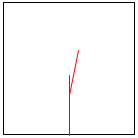They demonstrate how to generate a fractal Savannah tree in the same way that figure 156 shows how to draw a Sierpinski triangle. The image on the left shows what a fractal Savannah tree looks like. The right one explains the generative construction step.

Design the function add-savannah. The function consumes an image and four numbers: (1) the x-coordinate of a line’s base point, (2) the y-coordinate of a line’s base point, (3) the length of the line, and (4) the angle of the line. It adds a fractal Savannah tree to the given image.

Unless the line is too short, the function adds the specified line to the image. It then divides the line into three segments. It recursively uses the two intermediate points as the new starting points for two lines. The lengths and the angles of the two branches change in a fixed manner, but independently of each other. Use constants to define these changes and work with them until you like your tree well enough.

Hint Experiment with shortening each left branch by at least one third and rotating it left by at least 0.15 degrees. For each right branch, shorten it by at least 20% and rotate it by 0.2 degrees in the opposite direction.

Exercise 528. Graphics programmers often need to connect two points with a smooth curve where “smooth” is relative to some perspective.Géraldine Morin suggested this exercise. Here is are two sketches: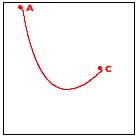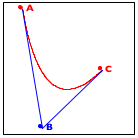The left one shows a smooth curve, connecting points A and C; the right one supplies the perspective point, B, and the angle of an observer.

One method for drawing such curves is due to Bézier. It is a prime example of generative recursion, and the following sequence explains the eureka! behind the algorithm: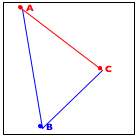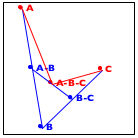Consider the image on the left. It reminds you that the three given points determine a triangle and that the connection from A to C is the focal point of the algorithm. The goal is to pull the line from A to C toward B so that it turns into a smooth curve.

Now turn to the image in the middle. It explains the essential idea of the generative step. The algorithm determines the midpoint on the two observer lines, A-B and B-C, as well as their midpoint, A-B-C.

Finally, the right-most image shows how these three new points generate two distinct recursive calls: one deals with the new triangle on the left and the other one with the triangle on the right. More precisely, A-B and B-C become the new observer points and the lines from A to A-B-C and from C to A-B-C become the foci of the two recursive calls.

When the triangle is small enough, we have a trivially solvable case. The algorithm just draws the triangle, and it appears as a point on the given image. As you implement this algorithm, you need to experiment with the notion of “small enough” to make the curve look smooth.

### 34Summary

This last part is about designing with accumulators, a mechanism for collecting knowledge during a data structure traversal. Adding an accumulator can fix performance flaws and eliminate termination problems. Your take away from this part are two and a half design lessons:
1. The first step is to recognize the need for introducing an accumulator. Traversals “forget” pieces of the argument when they step from one piece to the next. If you discover that such knowledge could simplify the function’s design, consider introducing an accumulator. The first step is to switch to the accumulator template.

2. The key step is to formulate an accumulator statement. The latter must express what knowledge the accumulator gathers as what kind of data. In most cases, the accumulator statement describes the difference between the original argument and the current one.

3. The third step, a minor one, is to deduce from the accumulator statement (a) what the initial accumulator value is, (b) how to maintain it during traversal steps, and (c) how to exploit its knowledge.

The idea of accumulating knowledge is ubiquitous, and it appears in many different forms and shapes. It is widely used in so-called functional languages like ISL+. Programmers using imperative languages encounter accumulators in a different way, mostly via assignment statements in primitive looping constructs because the latter cannot return values. Designing such imperative accumulator programs proceeds just like the design of accumulator functions here, but the details are beyond the scope of this first book on systematic program design.